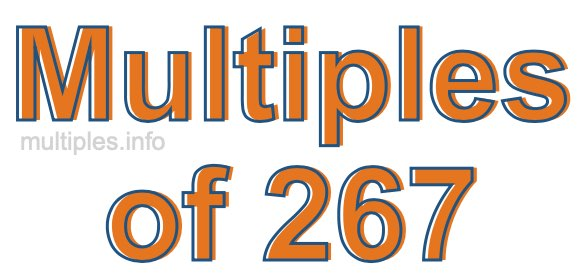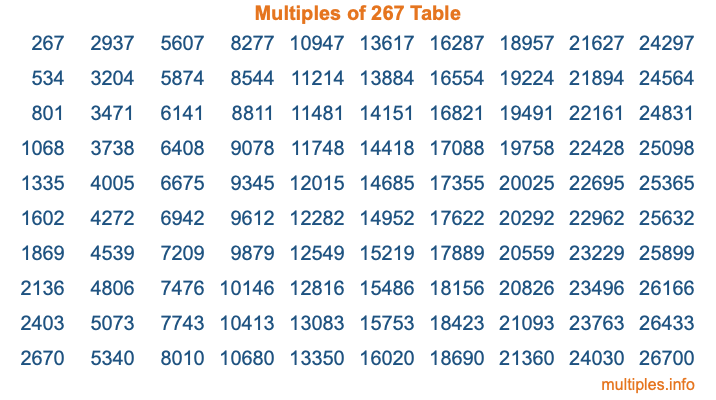Multiples of 267Welcome to the Multiples of 267 page. Here we will first teach you everything you will ever need to know about the multiples of 267, and then give you a study guide summary of everything we taught you to make sure you remember it all. Use this page to look up facts and learn information about the multiples of 267. This page will make you a multiples of two hundred sixty-seven expert!

Definition of Multiples of 267
Multiples of 267 are all the numbers that when divided by 267 equal an integer. Each of the multiples of 267 are called a multiple. A multiple of 267 is created by multiplying 267 by an integer.

Therefore, to create a list of multiples of 267, you start with 1 multiplied by 267, then 2 multiplied by 267, then 3 multiplied by 267, and so on for as long as you want. Thus, the list of the first five multiples of 267 is 267, 534, 801, 1068, and 1335. To see a larger list of multiples of 267, see the printable image of Multiples of 267 further down on this page. We also have a category where you can choose any nth multiple of 267.

Multiples of 267 Checker
The Multiples of 267 Checker below checks to see if any number of your choice is a multiple of 267. In other words, it checks to see if there is any number (integer) that when multiplied by 267 will equal your number. To do that, we divide your number by 267. If the the quotient is an integer, then your number is a multiple of 267.

Is  a multiple of 267?

Least Common Multiple of 267 and ...
A Least Common Multiple (LCM) is the lowest multiple that two or more numbers have in common. This is also called the smallest common multiple or lowest common multiple and is useful to know when you are adding our subtracting fractions. Enter one or more numbers below (267 is already entered) to find the LCM.

Check out our LCM Calculator if you need more details about the Least Common Multiple or if you need the LCM for different numbers for adding and subtraction fractions.

nth Multiple of 267
As we stated above, 267 is the first multiple of 267, 534 is the second multiple of 267, 801 is the third multiple of 267, and so on. Enter a number below to find the nth multiple of 267.

th multiple of 267

Multiples of 267 vs Factors of 267
267 is a multiple of 267 and a factor of 267, but that is where the similarities end. All postive multiples of 267 are 267 or greater than 267. All positive factors of 267 are 267 or less than 267.

Below is the beginning list of multiples of 267 and the factors of 267 so you can compare:

Multiples of 267: 267, 534, 801, 1068, 1335, etc.

Factors of 267: 1, 3, 89, 267

As you can see, the multiples of 267 are all the numbers that you can divide by 267 to get a whole number. The factors of 267, on the other hand, are all the whole numbers that you can multiply by another whole number to get 267.

It's also interesting to note that if a number (x) is a factor of 267, then 267 will also be a multiple of that number (x).

Multiples of 267 vs Divisors of 267
The divisors of 267 are all the integers that 267 can be divided by evenly. Below is a list of the divisors of 267.

Divisors of 267: 1, 3, 89, 267

The interesting thing to note here is that if you take any multiple of 267 and divide it by a divisor of 267, you will see that the quotient is an integer.

Multiples of 267 Table
Below is an image of the first 100 multiples of 267 in a table. The table is in chronological order, column by column. The first column has the first ten multiples of 267, the second column has the next ten multiples of 267, and so on.The Multiples of 267 Table is also referred to as the 267 Times Table or Times Table of 267. You are welcome to print out our table for your studies.

Negative Multiples of 267
Although not often discussed or needed in math, it is worth mentioning that you can make a list of negative multiples of 267 by multiplying 267 by -1, then by -2, then by -3, and so on, to get the following list of negative multiples of 267:

-267, -534, -801, -1068, -1335, etc.

Multiples of 267 Summary
Below is a summary of important Multiples of 267 facts that we have discussed on this page. To retain the knowledge on this page, we recommend that you read through the summary and explain to yourself or a study partner why they hold true.

There are an infinite number of multiples of 267.

A multiple of 267 divided by 267 will equal a whole number.

267 divided by a factor of 267 equals a divisor of 267.

The nth multiple of 267 is n times 267.

The largest factor of 267 is equal to the first positive multiple of 267.

267 is a multiple of every factor of 267.

267 is a multiple of 267.

A multiple of 267 divided by a divisor of 267 equals an integer.

267 divided by a divisor of 267 equals a factor of 267.

Any integer times 267 will equal a multiple of 267.

Multiples of a Number
Here you can get the multiples of another number, all with the same attention to detail as we did for multiples of 267 on this page.

Multiples of
Multiples of 268
Did you find our page about multiples of two hundred sixty-seven educational? Do you want more knowledge? Check out the multiples of the next number on our list!